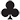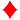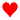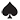Score for Making Contracts

Author: Larry Cohen
Date of publish: 07/14/2018
Level: Beginner to Intermediate

Note: There are 3 articles in this series. The start/cover page is here.

The scoring explained here is for Duplicate Bridge (matchpoints, teams, etc.).  Rubber bridge scoring is different.

Failing in a contract always results in a minus score, explained here.

For making any contract, there is a bonus (explained below). In addition, there is always a payout for each trick taken (assuming the contract makes).

Trick scores

If you make a contract, you've taken more than 6 tricks ("book"). All scoring starts with the 7th trick you take (the first 6 are assumed).  So, if you take 7 tricks, think of that as the starting point--thus "1".  If you take 9 tricks, you get awarded for "making 3."

In a making minor-suit contract (/), you get 20 per trick (starting with the 7th trick taken).  So, for taking 8 tricks in clubs ("making 2") you get 20 x 2=40. It doesn't matter whether you bid for 7 (1) or 8 (2); if you take 8 tricks, you get paid per trick whether they are overtricks or not.  Of course, if you bid 3and take only 8 tricks, you are down and get a minus score.

In a making Major-suit contract (/), you get 30 per trick.  So, for taking 8 tricks in hearts ("making 2") you get 30 x 2 = 60 (remember that the first 6 tricks taken don't count).

In a making Notrump contract (NT), you earn an extra 10 points above a Major contract. You get 30 per trick +10 extra.  So, for taking 9 tricks in notrump you get 30 x 3 +10 =100 (remember that the first 6 tricks taken don't count).

Bonuses

Every making contract also gets a bonus added. Here are the 4 kinds of contract:

Grand Slam = Bidding for all 13 tricks
Small Slam = Bidding for 12 tricks (you get the bonus if you make 12 or 13 tricks)
Game = Bidding for a # of tricks that adds to 100 or more (use the trick scores as explained above). The minimum threshold for game is 3NT (30 x 3 + 10 = 100) or 4/4(30 x 4 = 120) or 5/5(20 x 5 = 100)
Partscore = Bidding and making a contract less than game (less than 100 points bid for in trick score).

A bonus is never awarded unless you bid to the level indicated. If you bid 2 and make 7, you get only a partscore bonus.

What are the points awarded for the bonuses?

Partscores (no matter who is vulnerable "red") pay a 50-point bonus.

Games pay 300 not vulnerable and 500 vulnerable (but you don't also get the partscore bonus).

The bonus for bidding and making a slam also depends on vulnerability:

 Level bid Not Vul Bonus Vulnerable Bonus Small Slam 800 1250 Grand Slam 1300 2000

I've done something here you won't see in other scoring explanations. I've shown the actual bonus. Bidding and making a slam includes the game bonus. So, in a "traditional" chart, you'd see only 1500 for a vulnerable Grand Slam, but it also pays 500 since it is game, thus the 2,000 shown.  I think it is simpler this way.

All making contracts score the trick points and the bonus points.

The bonuses explained above are paid only if you bid to (and make) the level indicated.  If you make a small slam (12 tricks), you get the bonus only if you bid for 12 tricks.  If you make game, you get the bonus only if you bid to that level.

Examples (all of these contracts succeeded; if they are down, use this table).

Vulnerability always matters in the scoring if game or higher is bid.

 Contract Vulnerability Tricks taken Making How Many Trick Score Bonus Score 1irrelevant 8 2 2 x 20 = 40 +50= 90 2irrelevant 9 3 3 x 30 = 90 +50= 140 3NT Vul 10 4 4  x 30 + 10 = 130 +500= 630 4not vul 11 5 5 x 30 = 150 +300= 450 6not vul 13 7 7 x 20 = 140 +800= 940 7NT Vul 13 7 7 x 30 + 10 = 220 +2000= 2220